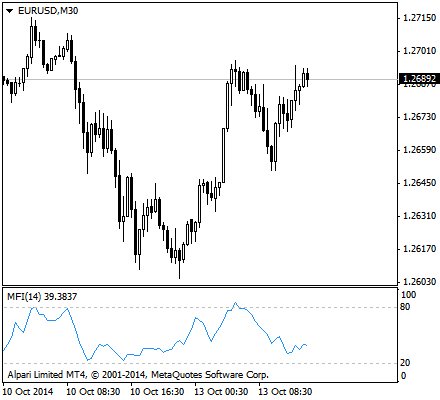# Using Money Flow Index to Trade Binaries

Here is yet another popular indicator that is used by many binary options traders. Like many indicators, the strategies that revolve around this indicator requires traders to have an in-depth understanding of the underlying factors that make it up. Calculations are needed, but today’s tools are already equipped with the functions of doing the calculations for the trader. However, it is still important for the binary options trader to know how the values were obtained.

The indicator that we shall discuss today is the Money Flow Index or MFI. MFI is an oscillator indicator that does not only use the price of an asset, but also its volume to measure buying and selling pressure. It is a momentum indicator, meaning it can denote price action movement and how quickly they change. The Money Flow Index is a standalone indicator, which means that traders can make informed decisions from the indicator alone.

MFI is very similar to the Relative Strength Index (RSI), which we have discussed in one of the previous articles. They are quite similar in both interpretation and calculation. MFI is a good measure of the strength of money flowing in and out of an asset, making it a rigid indicator. Because it is volume-weighted, it compares positive and negative money flows to identify the strength or weakness of a trend.

The Money Flow Index is therefore used to determine the conviction of a current trend. By analyzing the price and volume of a given asset, traders are able to know if the trend would be persistent, or a reversal is imminent. Because the MFI is used as a measure of the strength of money going in and out of a security, it can be used to predict a trend reversal. When traders detect this using MFI, they perform the appropriate actions.

The Money Flow Index was devised by by Gene Quong and Avrum Soudack. They intended MFI to be a volume-weighted RSI. The mechanics of MFI can be quite simple. It starts with the typical price for each trading period. Money flow is positive when the typical price rises, or when there is buying pressure, and negative when the typical price declines, or if there is a selling pressure. MFI shows these in terms of price and trading volume.

The ratio of positive and negative money flow is plugged into an RSI formula to create an oscillator that moves between 0 and 100. As a momentum oscillator tied to volume, the Money Flow Index is best used to identify reversals and price extremes with a variety of signals.
The MFI is often calculated using a 14 day period. Binary options traders can vary this period. All they need to do is to modify the period in the formula or in their chosen charting platform.

## Money Flow Index Calculation

There are a several steps involved in the Money Flow Index calculation where the following formulae are used.

First, the typical price is the average of the high, low, and closing prices:

Typical Price = (High + Low + Close) / 3

Then, the money flow is computed after obtaining the typical price value. The money flow is basically a monetary volume because the formula involves multiplying the volume by the typical price:

Money Flow = Typical price * Volume

Money Flow is positive when the typical price advances from one period to the next and negative when the typical price declines. Money flow values are not used when the typical price is unchanged.

The Money Ratio is the third step in the calculation and is the basis for the Money Flow Index (MFI). The Positive Money Flow sum is divided by the Negative Money Flow sum to create the ratio:

Money Ratio = Positive Money Flow / Negative Money Flow

Finally, the Relative Strength Index formula is then applied to create a volume-weighted indicator.

Money Flow Index = 100 — (100 / (1 + Money Ratio))

The positive money values are created if the current typical price is greater than the previous typical price value. The sum of positive money over the number of periods used to create the indicator of positive money flow. The opposite is true for the negative money flow values. After these two values are obtained, the ratio is taken and the formula for RSI is retained with the obtained money ratio plugged into the formula.

## MFI Interpretations

Because MFI is similar to RSI, they can interpreted similarly. MFI can signal divergences and overbought/oversold conditions. The MFI can be used as call or put signals comparing it to the positive and negative divergences of the price respectively.This is because MFI can often be used to indicate an imminent reversal of a trend. If a price value is falling, but positive money flow seems to be greater than negative money flow, then there is more volume associated with daily price rises that compensate the price drops. This may suggest a weak downtrend that threatens to reverse as money flowing into the asset is “stronger” than money flowing out of it.There are also other similarities of MFI and the RSI. As with the RSI, the MFI can also be used to determine if there is too much or too little volume associated with an asset. As with other indicators, a stock is considered overbought if the MFI indicator reaches a bearish 80 and above. On the other end of the spectrum, a bullish reading of 20 and below suggests a stock is oversold. Traders can now make appropriate actions based on this suggestions. A call option can be placed for oversold conditions and a put option can be placed for overbought conditions.

Because MFI is a volume-weighted version of RSI the big difference between the two is, of course, volume. Because volume is factored into the calculation of the indicator, the Money Flow Index may act a little differently than RSI. There are theories that suggest that volume leads prices. The RSI is a momentum oscillator that already leads prices. Incorporating volume using the MFI can increase this lead time.

Try out this indicator today. Select a top BO broker from the list we have compiled to start trading. Stay tuned for more helpful articles.

Read more articles on Education, Strategy.